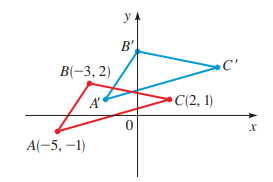# The new coordinates of the point ( 5 , 3 ) when the coordinate plane is shifted.### Precalculus: Mathematics for Calcu...

6th Edition
Stewart + 5 others
Publisher: Cengage Learning
ISBN: 9780840068071### Precalculus: Mathematics for Calcu...

6th Edition
Stewart + 5 others
Publisher: Cengage Learning
ISBN: 9780840068071

#### Solutions

Chapter 1.8, Problem 116E

(a)

To determine

## To calculate: The new coordinates of the point (5,3) when the coordinate plane is shifted.

Expert Solution

The new coordinates of the point (5,3) are (8,5) .

### Explanation of Solution

Given information:

All points in the coordinate in plane are shifted 3 units to the right and 2 units upwards.

Formula used:

If (x1,y1) and (x2,y2) are two pair of coordinates then (x1,y1)+(x2,y2)=(x1+x2,y1+y2) .

Calculation:

Consider the point (5,3) . It is provided that all points in the coordinate in plane are shifted 3 units to the right and 2 units upwards that is shifted by the pair (3,2) .

Recall that if (x1,y1) and (x2,y2) are two pair of coordinates then (x1,y1)+(x2,y2)=(x1+x2,y1+y2) .

So, the point (5,3) is shifted to,

(5,3)+(3,2)=(8,5)

Thus, the new coordinates of the point (5,3) are (8,5) .

(b)

To determine

### To calculate: The new coordinates of the point (a,b) when the coordinate plane is shifted.

Expert Solution

The new coordinates of the point (a,b) are (a+3,b+2) .

### Explanation of Solution

Given information:

All points in the coordinate in plane are shifted 3 units to the right and 2 units upwards.

Formula used:

If (x1,y1) and (x2,y2) are two pair of coordinates then (x1,y1)+(x2,y2)=(x1+x2,y1+y2) .

Calculation:

Consider the point (a,b) . It is provided that all points in the coordinate in plane are shifted 3 units to the right and 2 units upwards that is shifted by the pair (3,2) .

Recall that if (x1,y1) and (x2,y2) are two pair of coordinates then (x1,y1)+(x2,y2)=(x1+x2,y1+y2) .

So, the point (a,b) is shifted to,

(a,b)+(3,2)=(a+3,b+2)

Thus, the new coordinates of the point (a,b) are (a+3,b+2) .

(c)

To determine

### To calculate: The old coordinates of the point (3,4) when the coordinate plane is shifted.

Expert Solution

The old coordinates of the point (3,4) are (0,2) .

### Explanation of Solution

Given information:

All points in the coordinate in plane are shifted 3 units to the right and 2 units upwards.

Formula used:

If (x1,y1) and (x2,y2) are two pair of coordinates then (x1,y1)+(x2,y2)=(x1+x2,y1+y2) .

Calculation:

Consider the point (3,4) . It is provided that all points in the coordinate in plane are shifted 3 units to the right and 2 units upwards that is shifted by the pair (3,2) .

Recall that if (x1,y1) and (x2,y2) are two pair of coordinates then (x1,y1)+(x2,y2)=(x1+x2,y1+y2) .

So, the point (3,4) was originally at, let that point be (x,y)

(x,y)+(3,2)=(3,4)(x,y)=(3,4)(3,2)=(33,42)=(0,2)

Thus, the old coordinates of the point (3,4) are (0,2) .

(d)

To determine

### To calculate: The new coordinates of the points A,B and C when the coordinate plane is shifted.Expert Solution

The new coordinates of the points A,B and C are A'=(2,1),B'=(0,4) and C'=(5,3) .

### Explanation of Solution

Given information:

All points in the coordinate in plane are shifted 3 units to the right and 2 units upwards.

Formula used:

If (x1,y1) and (x2,y2) are two pair of coordinates then (x1,y1)+(x2,y2)=(x1+x2,y1+y2) .

Calculation:

Consider the triangle ABC shifted to triangle A'B'C' .It is provided that all points in the coordinate in plane are shifted 3 units to the right and 2 units upwards that is shifted by the pair (3,2) .

Recall that if (x1,y1) and (x2,y2) are two pair of coordinates then (x1,y1)+(x2,y2)=(x1+x2,y1+y2) .

So, the point A(5,1) is shifted to,

(5,1)+(3,2)=(2,1)

So, the point B(3,2) is shifted to,

(3,2)+(3,2)=(0,4)

So, the point C(2,1) is shifted to,

(2,1)+(3,2)=(5,3)

Thus, the new coordinates of the points A,B and C are A'=(2,1),B'=(0,4) and C'=(5,3) .

### Have a homework question?

Subscribe to bartleby learn! Ask subject matter experts 30 homework questions each month. Plus, you’ll have access to millions of step-by-step textbook answers!